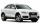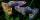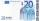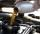Ratio

6 numbers are in the ratio 1:5:1:5:5:5. Their sum is 242. What are the numbers?

Result

a =  11
b =  55
c =  11
d =  55
e =  55
f =  55

Solution:Leave us a comment of example and its solution (i.e. if it is still somewhat unclear...):Be the first to comment!To solve this example are needed these knowledge from mathematics:

Do you have a system of equations and looking for calculator system of linear equations?

Next similar examples:

1. Ratio three numbersThree numbers SUV are in the ratio 1:2:3. Their sum is 24. Find this numbers and write their add and sum.
2. Two numbersFind two numbers whose difference and ratio is 2.
3. RatiosReduce the numbers: 50 in a 1:2 ratio 111 at a ratio of 2:3 70 at 10:50 560 at a ratio of 3:8
4. PeppersIn the box are yellow (a), green (b) and red (c) peppers. Their amount is in a ratio 2:4:1 . Most are yellow peppers and green the least. Calculate the number of peppers each type if the total number of peppers is 70.
5. AgesJohn, Teresa, Daniel and Paul have summary 56 years. Their ages are in a ratio of 1:2:5:6. Determine how many years have each of them.
6. Moneys in triple ratioMilan, John and Lili have a total 344 euros. Their amounts are in the ratio 1:2:5. Determine how much each of them has?
7. Ounce749 calories in a 7 - ounce serving . What is the unit rate?
8. GuppiesAudrey has some guppies in a fish tank. The ration of the oranges guppies to silver guppies is 3:5. She has 12y oranges guppies. Write the number of silver guppies she has in terms of y
9. Money splitAnton and Ferko got 2,500 euros together. The amount was split in 1:4 ratio. How much did each get?
10. Temperature 5Temperature outside starts at 0 Fahrenheit. Over time the temperature changes at a rate of -0.6 Fahrenheit per hour. How long will it take for the temperature to reach -4.5 Fahrenheit?
11. RunnersFor three runners (on the first to third place) is prepared 30 chocolate that they be distributed in the ratio of 3 : 2 : 1 How much chocolate will get everyone?
12. Divide moneyDivide 1200 USD at a ratio of 1:2:3:4:5:6:9:10
13. BuildingAt the building, we divided 240 boards into two piles in a 5: 3 ratio. How many were fewer boards in the lower pile?
14. QuizTested student answered correctly on a 4/ 8 questions. Wrong answers was 16. How many questions answered?
15. Motor oil30 liters of oil cost 28.80 Euros. How much cost a liter?
16. CustomerA customer purchase three (3) writing pads from a store. She receive k9.70 change from a k10.00 note . How much was it's writing pad cost?
17. HostelStudents are accommodated in 22 rooms. Rooms were 4 and 6 bed. How many rooms in which type occupied 106 children there?